SYLLABUS  Previous: 5.3.1 The Vasicek model  Up: 5.3 Methods for bonds  Next: 5.4 Computer quiz

5.3.2 Extensions for derivatives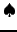[ SLIDE swap - cap-/floorlet - option || VIDEO modem - LAN - DSL]

Looking at a swap as being a bond paying a coupon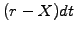over an infinitesimally short time interval, the differential change in the value of a bond (3.5#eq.6) can simply be supplemented with the corresponding increment. This immediately leads to the partial differential equation for a swap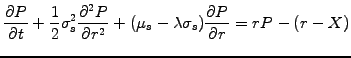(5.3.2#eq.1)

and can again be solved using the finite element method. The new term does not involve any unknown and therefore appears on the right hand side of the linear problem (5.3.1#eq.4) with a contribution that can be integrated analytically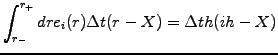(5.3.2#eq.2)

It has been coded into FEMSolution.java with an increment of the right hand side vector
// Construct the problem
c=b.dot(f);                                      //  A*fp=B*f=c as before
for (int i=1; i<n; i++)                          // Add swap source term
c[i]+= timeStep*h*(i*h-X);
c0=(f+(x-X)*timeStep)*Math.exp(timeStep*X);// Bounday conditions
a.setL(0, 0.);a.setD(0, 1.);a.setR(0, 0.);c=c0;//left: Dirichlet
dPdyn=(f[n]-f[n-1])/h; cn=c[n-1]-2*dx*a.getL(n-1)*dPdyn;
a.setL(n,a1n);a.setD(n,ann);a.setR(n, 0.);c[n]=cn;//right:Neuman
The Dirichlet boundary condition has been modified to account for the compounded interest from the fixed swap rate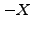, but the rest remains the same as for the pricing of a bond.
Similar considerations are valid for caplets and floorlets: viewed as a bond paying a coupon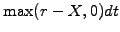for the caplet and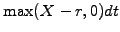for the floorlet, this yields the modified Vasicek equation for a caplet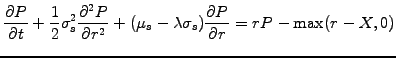(5.3.2#eq.3)

and, by replacing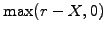with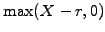, the counterpart for the floorlet. Both have been implemented into FEMSolution.java and the scheme for the caplet reads
// Construct the problem
c=b.dot(f);                                      //  A*fp=B*f=c as before
for (int i=1; i<n; i++)
if (i*h<X) c[i]+=0;
else       c[i]+=timeStep*h*(i*h-X);
a.setL(0, 0.);a.setD(0, 1.);a.setR(0, 0.);c=0.;//left: Dirichlet
dPdyn=0; cn=c[n-1]-2*dx*a.getL(n-1)*dPdyn;
a.setL(n,a1n);a.setD(n,ann);a.setR(n, 0.);c[n]=cn;//right:Neuman
Having calculated the fair price for a bond, a swap, cap or floor, it is relatively easy to calculate the value of derivatives such as bond options, swaptions, captions and floortions: their value depends on the same random variable and therefore satisfies the same equation as the underlying. For example, after calculating the value of the bond by solving the Vasicek equation (3.5#eq.6) backwards in time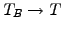, the terminal bond option payoff (2.2.4#eq.1) can be used to integrate backwards further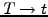until the present value of the bond option is found.

SYLLABUS  Previous: 5.3.1 The Vasicek model  Up: 5.3 Methods for bonds  Next: 5.4 Computer quiz